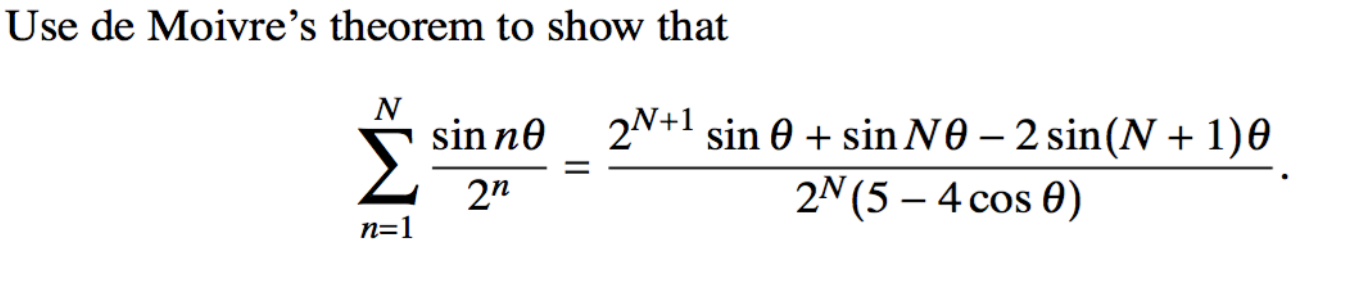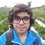# Complex Numbers Help needed.I tried the following approach.

Let $$z = \dfrac{ e^{ i \theta }}{2}$$. Then using de Moivre's theorem:

$\sum _ { n = 1 } ^ { N } \frac{ \sin n \theta } { 2 ^ n } = \text { Im } \left ( \sum _ { n = 1 } ^ { N } z^ { n} \right)$

This is sum of GP. Using the sum of GP:

$\sum _{ n = 1 } ^{ N } z^ { n} = \frac{ \frac{e ^{ i \theta } } { 2 } ( 1 - \frac{e ^{ N i \theta } } {2^ { N } } ) } { 1- \frac{ e^{i \theta } } { 2 } }$

This simplifies to:

$\sum _{ n = 1 } ^{ N } z^ { n} = \frac{ e ^{ i \theta } ( 2 ^ { N } - e ^{ N i \theta } ) } { 2 ^ { N} ( 2- e^{i \theta } ) }$

$\sum _{ n = 1 } ^{ N } z^ { n} = \frac{ ( 2 ^ { N } e ^{ i \theta } - e ^{ (N + 1 ) i \theta } ) } { 2 ^ { N} ( 2- e^{i \theta } ) } ~~~~~ (*)$

Now I changed the complex numbers on the RHS from Euler's form to cis form, then made the denominator real and finally took imaginary part of the expression. Is there a simpler way to solve this problem? My method is very complicated, thus error-prone.

In fact, I have already made an error which is why I have posted this note. I am getting the RHS as

$\frac{2 ^ { N + 1 } \sin \theta - 2 \sin (N + 1) \theta } {2 ^ N (5 - 4 \cos \theta) }$

i.e. without the $\sin N \theta$ term in the numerator. Where is the $\sin N \theta$ term coming from? There are only $e^{ i \theta }$ and $e ^{ (N + 1 ) i \theta }$ in the $(*)$ equation. I can't seem to find out where I have gone wrong.

Thanks.

###### Source: CIE A Levels Further Maths May / June 2007 Q 11 (b)Note by Pranshu Gaba
5 years, 10 months ago

This discussion board is a place to discuss our Daily Challenges and the math and science related to those challenges. Explanations are more than just a solution — they should explain the steps and thinking strategies that you used to obtain the solution. Comments should further the discussion of math and science.

When posting on Brilliant:

• Use the emojis to react to an explanation, whether you're congratulating a job well done , or just really confused .
• Ask specific questions about the challenge or the steps in somebody's explanation. Well-posed questions can add a lot to the discussion, but posting "I don't understand!" doesn't help anyone.
• Try to contribute something new to the discussion, whether it is an extension, generalization or other idea related to the challenge.

MarkdownAppears as
*italics* or _italics_ italics
**bold** or __bold__ bold
- bulleted- list
• bulleted
• list
1. numbered2. list
1. numbered
2. list
Note: you must add a full line of space before and after lists for them to show up correctly
paragraph 1paragraph 2

paragraph 1

paragraph 2

[example link](https://brilliant.org)example link
> This is a quote
This is a quote
    # I indented these lines
# 4 spaces, and now they show
# up as a code block.

print "hello world"
# I indented these lines
# 4 spaces, and now they show
# up as a code block.

print "hello world"
MathAppears as
Remember to wrap math in $$ ... $$ or $ ... $ to ensure proper formatting.
2 \times 3 $2 \times 3$
2^{34} $2^{34}$
a_{i-1} $a_{i-1}$
\frac{2}{3} $\frac{2}{3}$
\sqrt{2} $\sqrt{2}$
\sum_{i=1}^3 $\sum_{i=1}^3$
\sin \theta $\sin \theta$
\boxed{123} $\boxed{123}$

Sort by:

I think you are missing out any term. I solved the RHS and got the desired result. Recheck it. As far as i think this is a proofing problem and the method you followed is very simple and short i think. Just need some care while solving.

- 5 years, 9 months ago

Thanks Sachin :), I realized I was silly mistakes. I solved it again carefully and now I got the desired result.

- 5 years, 9 months ago

take $sin(x)=\dfrac{-i}{2}(e^{ix}-e^{-ix}$. show this is true by de moivres.

- 5 years, 9 months ago

Thanks for helping Aareyan, this method is nice! :)

- 5 years, 9 months ago